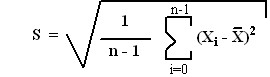Previous  Top  Next

# Windows Time Client

Standard Deviation Statistical Filter

Standard Deviation is a statistical measure of variance in a sample set. It is calculated from the square root of the variance of the sample set as shown below :where n is the number of data samples
anddenotes the arithmetic mean of the sample set.

The standard deviation of time measurements is important to PresenTense Time Client because it reflects the quality of the network connection to the legal source of Coordinated Universal Time and therefore reflects the quality of the time measurements themselves. As the quality of service on the internet is subject to variation, the standard deviation is computed for every set of measurements. If the standard deviation is excessively large or varies substantially from previous measurements, the affected measurements are marked as discarded.